### 2016-06-14

pcadapt has been developed to detect genetic markers involved in biological adaptation. pcadapt provides statistical tools for outlier detection based on Principal Component Analysis (PCA).

In the following, we show how the pcadapt package can perform genome scans for selection. The package contains two examples: geno3pops (genotype data) and pool3pops (Pool-seq data). The geno3pops data contain simulated genotypes for 1,500 diploid markers. A total of 150 individuals coming from three different populations were genotyped. Simulations were performed with simuPOP using a divergence model assuming that 200 SNPs confer a selective advantage. The pool3pops data contain simulated allele frequencies in 3 populations for 1,500 diploid markers. Allele frequencies have been computed based on the geno3pops dataset.

To run the package, you need to install it and to load it using the following command lines:

install.packages("pcadapt")
library(pcadapt)

The main change of the 3.0 version is that all analyses are now included in the R package. Users should not use the C software PCAdapt fast anymore.

# A. Reading the genotype data

## A.1. The read.pcadapt function

You should use the read.pcadapt function to convert your genotype file to the pcadapt format. pcadapt files should have individuals in columns, SNPs in lines, and missing values should be encoded with 9. read.pcadapt converts different types of files to the pcadapt format and returns a character string containing the name of the converted file, which should be used as input for the pcadapt function. The current version of read.pcadapt supports three formats: “vcf”, “ped”, “lfmm” and “pcadapt”. For example, assume your genotype file is called “foo.lfmm” and is located in the directory “path_to_directory”, use the following command lines:

path_to_file <- "path_to_directory/foo.lfmm"
print(filename)

NB: the lfmm format was the former format used for older versions of pcadapt.

To run the provided example, the file location can be retrieved by typing the following command:

path_to_file <- system.file("extdata","geno3pops",package="pcadapt")

## A.2. How to handle missing values

As stated above, to proceed with the pcadapt function, files should be in the right format. Any missing value should be encoded with a 9. This is automatically done with read.pcadapt if it has been used to convert “ped”, “vcf” or “lfmm” files.

# B. Choosing the number K of Principal Components

The pcadapt function performs two successive tasks. First, PCA is performed on the centered and scaled genotype matrix. The second stage consists of computing test statistics and p-values based on the correlations between SNPs and the first K principal components (PCs). To run the function pcadapt, the user should specify the pcadapt file location filename or use the output returned by the function read.pcadapt, along with the number K of principal components to work with.

To choose K, principal component analysis should first be performed with a large enough number of principal components (e.g. K=20).

x <- pcadapt(filename,K=20,transpose=TRUE)

NB : by default, data are assumed to be diploid. To specify the ploidy, use the argument ploidy (ploidy=2 for diploid species and ploidy=1 for haploid species) in the pcadapt function.

## B.1. Scree plot

The ‘scree plot’ displays in decreasing order the percentage of variance explained by each PC. Up to a constant, it corresponds to the eigenvalues in decreasing order. The ideal pattern in a scree plot is a steep curve followed by a bend and a straight line. The eigenvalues that correspond to random variation lie on a straight line whereas the ones that correspond to population structure lie on a steep curve. We recommend to keep PCs that correspond to eigenvalues to the left of the straight line. In the provided example, K=2 is the optimal choice for K. The plot function displays a scree plot:

plot(x,option="screeplot")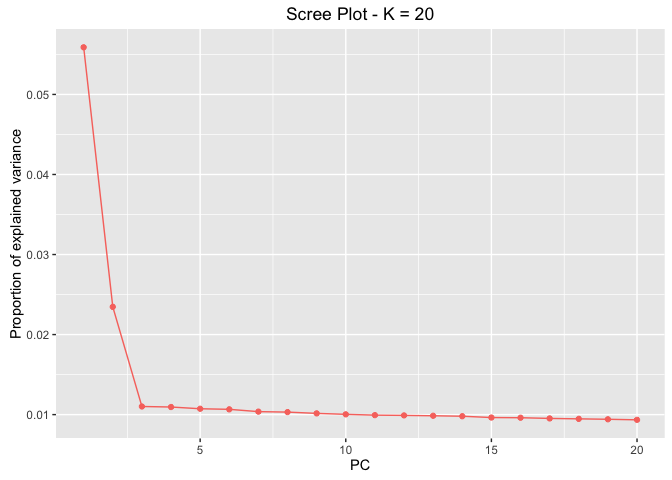By default, the number of principal components taken into account in the scree plot is set to K, but it can be reduced via the argument K.

plot(x,option="screeplot",K=10)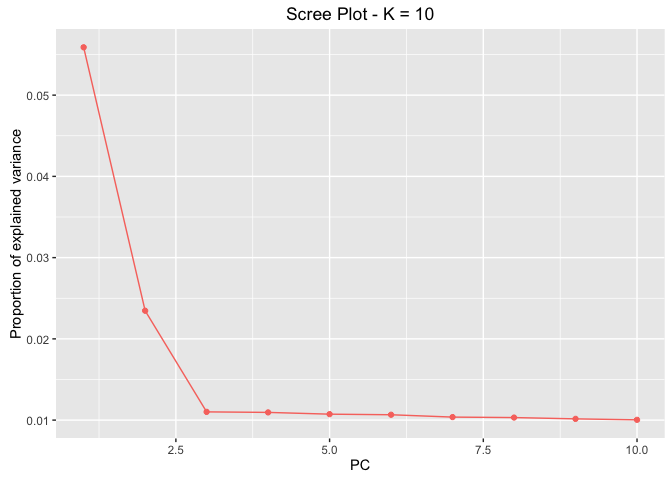## B.2. Score plot

Another option to choose the number of PCs is based on the ‘score plot’ that displays population structure. The score plot displays the projections of the individuals onto the specified principal components. Using the score plot, the choice of K can be limited to the values of K that correspond to a relevant level of population structure.

When population labels are known, individuals of the same populations can be displayed with the same color using the pop argument, which should contain the list of indices of the populations of origin. In the geno3pops example, the first population is composed of the first 50 individuals, the second population of the next 50 individuals, and so on. Thus, a vector of indices or characters (population names) that can be provided to the argument pop should look like this:

poplist <- c(rep(1,50),rep(2,50),rep(3,50))
print(poplist)
##    1 1 1 1 1 1 1 1 1 1 1 1 1 1 1 1 1 1 1 1 1 1 1 1 1 1 1 1 1 1 1 1 1 1 1
##   1 1 1 1 1 1 1 1 1 1 1 1 1 1 1 2 2 2 2 2 2 2 2 2 2 2 2 2 2 2 2 2 2 2 2
##   2 2 2 2 2 2 2 2 2 2 2 2 2 2 2 2 2 2 2 2 2 2 2 2 2 2 2 2 2 2 3 3 3 3 3
##  3 3 3 3 3 3 3 3 3 3 3 3 3 3 3 3 3 3 3 3 3 3 3 3 3 3 3 3 3 3 3 3 3 3 3
##  3 3 3 3 3 3 3 3 3 3

If this field is left empty, the points will be displayed in black. By default, if the values of i and j are not specified, the projection is done onto the first two principal components.

plot(x,option="scores",pop=poplist)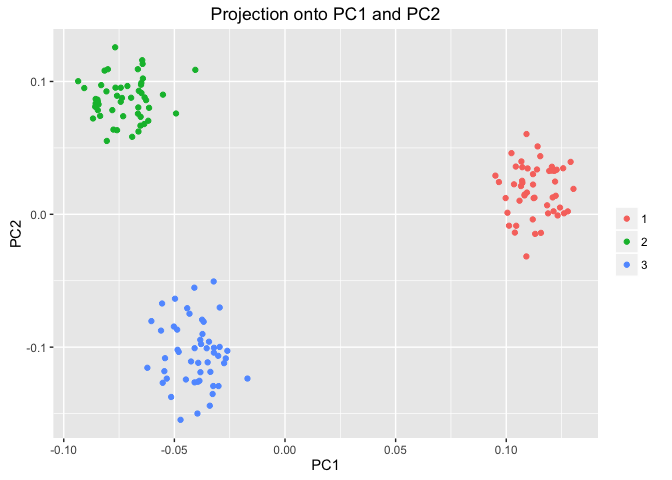Looking at population structure beyond K=2 confirms the results of the scree plot. The third and the fourth principal components do not ascertain population structure anymore.

plot(x,option="scores",i=3,j=4,pop=poplist)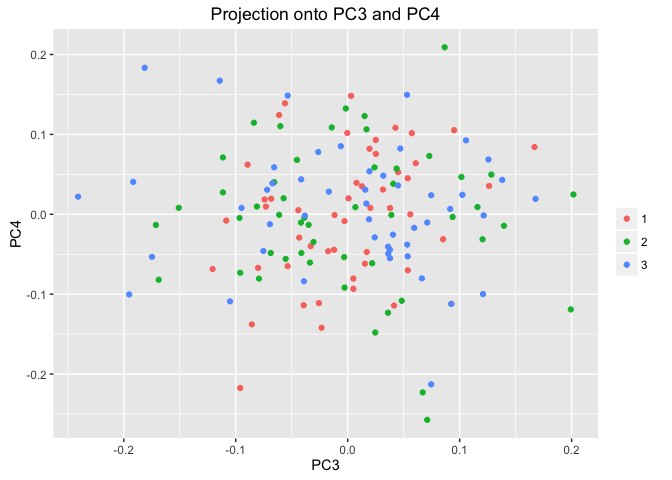NB: for .ped files, the plot function adds colors to the ‘score plot’ automatically provided that the data have been loaded with read.pcadapt.

# C. Computing the test statistic based on PCA

For a given SNP, the test statistic is based on the $$z$$-scores obtained when regressing SNPs with the K principal components. The test statistic for detecting outlier SNPs is the Mahalanobis distance, which is a multi-dimensional approach that measures how distant is a point from the mean. Denoting by $$z^j = (z_1^j, \dots, z_K^j)$$ the vector of K $$z$$-scores between the $$j$$-th SNP and the first K PCs, the sqaured Mahalanobis distance is defined as

$D_j^2 = (z^j - \bar{z})\Sigma^{-1}(z^j - \bar{z})$

where $$\bar{z}$$ and $$\Sigma$$ are robust estimates of the mean and of the covariance matrix. Once divided by a constant $$\lambda$$ called the genomic inflation factor, the scaled squared distances $$D_j^2/\lambda$$ should have a chi-square distribution with K degrees of freedom under the assumption that there are no outlier.

For the geno3pops data, it was found in section B that K=2 corresponds to the optimal choice of the number of PCs.

x <- pcadapt(filename,K=2)

In addition to the number K of principal components to work with, the user can also set the parameter min.maf that corresponds to a threshold of minor allele frequency. By default, the parameter min.maf is set to 5%. P-values of SNPs with a minor allele frequency smaller than the threshold are not computed (NA is returned).

The object x returned by the function pcadapt contains numerical quantities obtained after performing a PCA on the genotype matrix.

summary(x)

We assume in the following that there are n individuals and L markers.

• stat is a vector of size L containing squared Mahalanobis distances by default.

• pvalues is a vector containing L p-values.

• maf is a vector of size L containing minor allele frequencies.

• gif is a numerical value corresponding to the genomic inflation factor estimated from stat.

• chi2.stat is a vector of size L containing the rescaled statistics stat/gif that follow a chi-squared distribution with K degrees of freedom.

• scores is a (n,K) matrix corresponding to the projections of the individuals onto each PC.

• loadings is a (L,K) matrix containing the correlations between each genetic marker and each PC.

• singular.values is a vector containing the K ordered squared root of the proportion of variance explained by each PC.

• zscores is a (L,K) matrix containing the $$z$$-scores.

All of these elements are accessible using the $ symbol. For example, the p-values are contained in x$pvalues.

# D. Graphical tools

## D.1. Manhattan Plot

A Manhattan plot displays $$-\text{log}_{10}$$ of the p-values.

plot(x,option="manhattan")

## D.2. Q-Q Plot

The user is also given the possibility to check the distribution of the p-values using a Q-Q plot

plot(x,option="qqplot",threshold=0.1)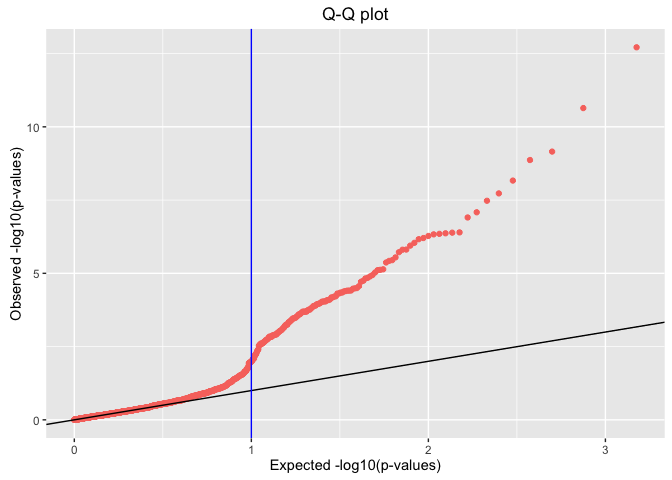On the right side of the grey dotted line are the top 10% lowest p-values. This plot confirms that most of the p-values follow the expected uniform distribution. However, the smallest p-values are smaller than expected confirming the presence of outliers.

## D.2. Histograms of the test statistic and of the p-values

A histogram of p-values confirms that most of the p-values follow the uniform distribution, and that the excess of small p-values indicates the presence of outliers.

hist(x$pvalues,xlab="p-values",main=NULL,breaks=50)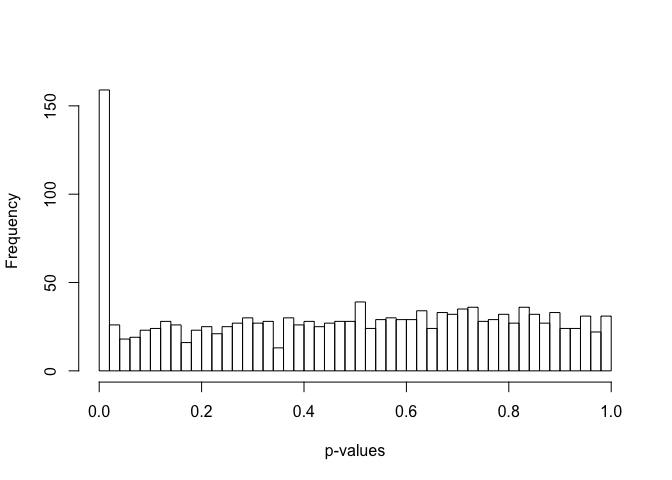The presence of outliers is also visible when plotting a histogram of the test statistic $$D_j$$. plot(x,option="stat.distribution")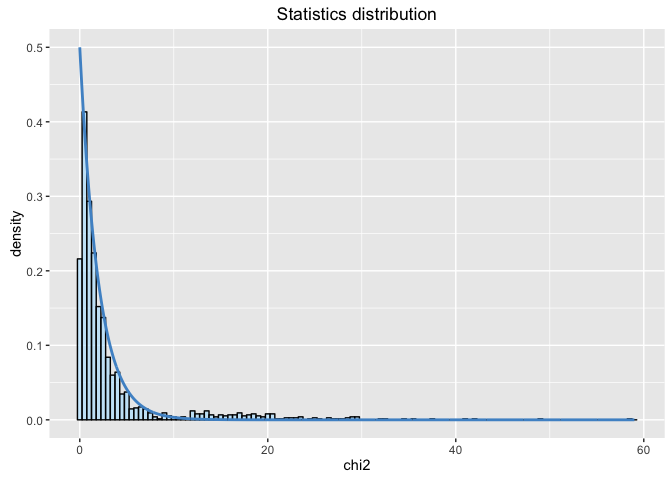# E. Choosing a cutoff for outlier detection To provide a list of outliers, we recommend using the R package qvalue, transforming the p-values into q-values. To install and load the package, type the following command lines: ## try http if https is not available source("https://bioconductor.org/biocLite.R") biocLite("qvalue") library(qvalue) For a given $$\alpha$$ (real valued number between $$0$$ and $$1$$), SNPs with q-values less than $$\alpha$$ will be considered as outliers with an expected false discovery rate bounded by $$\alpha$$. The false discovery rate is defined as the percentage of false discoveries among the list of candidate SNPs. Here is an example of how to provide a list of candidate SNPs for the geno3pops data, for an expected false discovery rate lower than 10%: qval <- qvalue(x$pvalues)$qvalues alpha <- 0.1 outliers <- which(qval<alpha) print(outliers) ##  1 2 3 4 5 6 7 8 10 11 12 13 15 16 ##  17 18 21 22 23 24 25 26 27 28 29 30 31 32 ##  33 34 36 37 38 39 40 41 42 43 44 45 46 47 ##  48 50 51 52 53 54 55 56 57 58 59 60 61 62 ##  63 64 65 66 67 69 70 71 72 73 74 75 76 77 ##  78 79 80 81 82 83 84 85 86 87 88 89 90 91 ##  92 93 94 95 97 98 99 100 101 102 103 104 105 106 ##  107 110 111 112 113 114 115 116 117 118 119 120 121 122 ##  123 124 125 126 127 128 129 130 131 132 133 134 135 137 ##  138 139 140 141 142 143 144 145 146 147 148 149 150 221 ##  262 414 425 441 525 526 619 625 663 932 1238 1368 1399 1406 It may be interesting to know which principal components are actually the most correlated with the oulier SNPs. The function get.pc allows to achieve that: snp_pc <- get.pc(x,outliers) head(snp_pc) ## SNP PC ## [1,] 1 1 ## [2,] 2 1 ## [3,] 3 1 ## [4,] 4 1 ## [5,] 5 1 ## [6,] 6 1 # F. Pool-seq Data The package also handles Pool-seq data. A Pool-seq example is provided in the package and can be loaded as follows: pooldata <- system.file("extdata","pool3pops",package="pcadapt") The frequency matrix should have n rows and L columns by default (where n is the number of populations and L is the number of genetic markers). If the input data is a (L,n) matrix, set transpose to TRUE in the pcadapt function. When calling the pcadapt function, make sure to specify data.type ="pool". xpool <- pcadapt(pooldata,data.type="pool",transpose=FALSE) ## Number of SNPs: 1500 ## Number of populations: 3 As for genotype data, the pcadapt function performs two successive tasks. First PCA is performed on the centered matrix of allele frequencies (not scaled). And the second stage consists of computing test statistics (Mahalanobis distances by default) and p-values based on the covariances between allele frequencies and the first K PCs. By default, the function assumes that the number K of PCs is equal to the number of populations minus one (K=n-1). The user can use a smaller number of PCs (K < n-1) by determining the optimal number of PCs using the scree plot. Plotting options mentioned in section D such as "manhattan", "qqplot", "scores", "screeplot", or "stat.distribution" are still valid for Pool-seq data. # G. Advanced Usage ## G.1 Reading data already loaded If your L x n genotype matrix data is already stored in the environment and is in the right format (pcadapt files should have individuals in columns, SNPs in lines, and missing values should be encoded with 9), you can run pcadapt directly using the following command line: data <- read.table(path_to_file) x <- pcadapt(data,K=2) If your genotype matrix is n x L, it is necessary to tranpose the matrix via the option transpose, a logical value indicating whether the matrix has to be transposed or not: data <- read.table(path_to_file) x <- pcadapt(data,K=2,transpose=TRUE) We recommend to check that pcadapt has been run correctly by verifying that the number of detected loci displayed in the console when running pcadapt, is correct. ## G.2 Computing other test statistics The default option uses the Mahalanobis distance as a test statistic to seek for outlier SNPs, but other statistics can be computed based on the correlations between SNPs and principal components. However except for power users, we recommend to use the Mahalanobis distance that provides the best rankings of SNPs in most cases we have investigated. ### G.2.1 Communality The communality statistic measures the proportion of variance explained by the first K PCs. When there are K+1 populations, the communality statistic provides a ranking similar to the widely used Fst statistic. P-values are computed using a chi-square approximation (Duforet-Frebourg et al. 2015). The test based on the communality statistic can be performed by setting method to communality in the pcadapt function. x_com <- pcadapt(filename,K=2,method="communality") The communality can be approximated by a constant times a chi-square distribution, allowing to compute p-values for each SNP. plot(x_com,option="stat.distribution")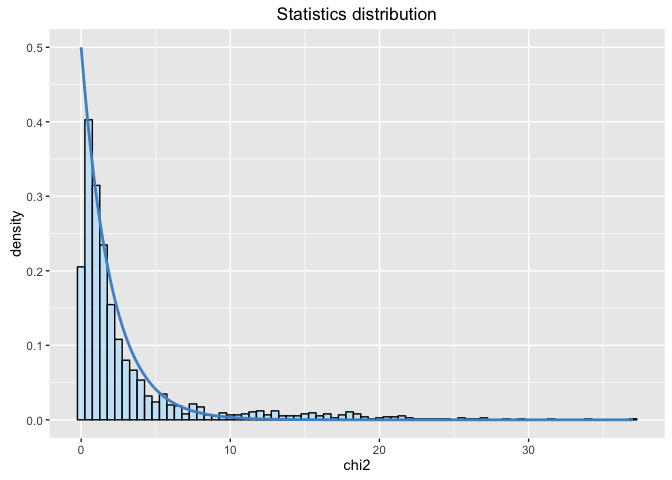### G.2.2 Component-wise Another possibility (component-wise p-values) is to perform one genome scan for each principal component. The test statistics are the loadings, which correspond to the correlations between each PC and each SNP. P-values are computed by making a Gaussian approximation for each PC and by estimating the standard deviation of the null distribution. x_cw <- pcadapt(filename,K=2,method="componentwise") summary(x_cw$pvalues)
##        V1               V2
##  Min.   :0.0000   Min.   :0.0000
##  1st Qu.:0.1434   1st Qu.:0.3259
##  Median :0.4229   Median :0.5724
##  Mean   :0.4322   Mean   :0.5514
##  3rd Qu.:0.6882   3rd Qu.:0.7971
##  Max.   :0.9999   Max.   :0.9995

pcadapt returns K vectors of p-values (one for each principal component), all of them being accessible, using the $ symbol or the [] symbol. For example, typing x_cw$pvalues$p2 or x_cw$pvalues[,2] in the R console returns the list of p-values associated with the second principal component (provided that K is larger than or equal to 2).

The p-values are computed based on the matrix of loadings. The loadings of the neutral markers are assumed to follow a centered Gaussian distribution. The standard deviation of the Gaussian distribution is estimated using the median absolute deviation.

To display the neutral distribution for the component-wise case, the value of K has to be specified.

plot(x_cw,option="stat.distribution",K=2)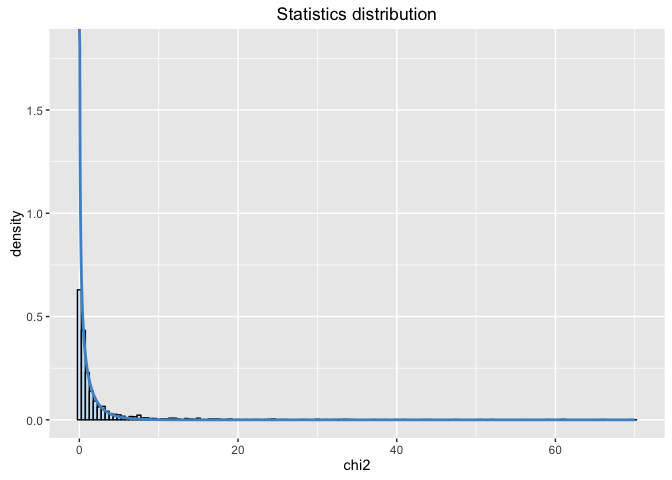## Changelog pcadapt 3.0.2 (06-13-2016)

• The function get.pc has been added. For each SNP, it returns the most correlated principal component.

## Changelog pcadapt 3.0.1 (06-01-2016)

• The read4pcadapt is now deprecated, it is now called read.pcadapt.
• Using the pop option when plotting scores now provides the color legend.
• The function get.pc has been added. See above for details.

## Changelog pcadapt 3.0 (04-04-2016)

• All analyses are now included in the R package. Users should not use the C software PCAdapt fast anymore.
• Big datasets can be handled directly within the R session.
• read4pcadapt now converts files to the pcadapt format.
• The first argument of pcadapt can be either a small genotype matrix or the output of read4pcadapt.

## Changelog pcadapt 2.2 (02-12-2016)

• The Mahalanobis distance is now estimated from the z-scores rather than the loadings.

## Changelog pcadapt 2.1 (12-18-2015)

• The scaling of the SNP before computing PCA has been changed. Instead of using standard deviation, we now use the square root of $$p(1-p)$$ (haploid data) or of $$2p(1-p)$$ (diploid data) where $$p$$ is the minimum allele frequency.

• Bug fix: the genomic inflation factor has been corrected when K=1.

• Bug fix: a problem due to high proportion of missing data slowing the program has been fixed.

• Argument "minmaf" has been replaced with "min.maf".

## Changelog pcapdapt 2.0.1

• Vignette corrected: when reading output from the software PCAdapt, we do not mention the deprecated argument PCAdapt in the function pcadapt.

• Bug fix: an issue occurring when reading outputs from PCAdapt has been fixed.

• We mention in the vignette how to use Pool-seq data with the C software PCAdapt fast.

## Changelog pcadapt 2.0

• The default test statistic is not the communality statistic anymore but the Mahalanobis distance.

• Test statistic for Pool-seq data.

# Reference

Luu, K., Bazin, E., & Blum, M. G. (2016). pcadapt: an R package to perform genome scans for selection based on principal component analysis.